Next: LEVINSON RECURSION Up: Waveform applications of least Previous: ADAPTIVE FILTERS

# DESIGN OF MULTICHANNEL FILTERS

Multichannel filters are frequently useful. For example, with a vector-prediction filter one might wish to predict a time series, using its past and the past of a group of other series. With a matrix-prediction filter one could predict a group of series, using the past of the whole group. If the series are related, the group prediction should be better than self-prediction of individual channels. For definiteness, let us take two time series xt and yt and suppose we are to find a vector filter which converts them into a third series dt. If dt is xt+1, this is a unit time-span prediction for filter for xt. If dt is a vertical seismogram and xt and yt are horizontals, then the two-channel filter might be called an extrapolation filter. The set of equations which we wish to solve by least squares takes the form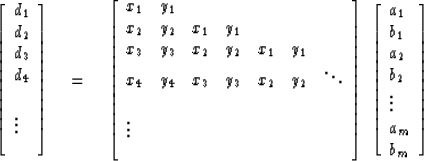(29)

If this set of equations is abbreviated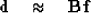(30)

then, as we have seen in an earlier chapter, the solution is of the form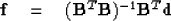(31)

We wish to inspect the matrix being inverted, call it R. For a filter with three time lags we get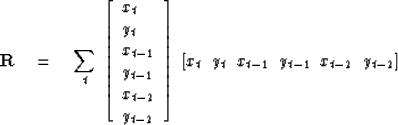(32)

If we define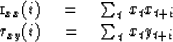and likewise for ryx(i) and ryy(i) the matrix (32) becomes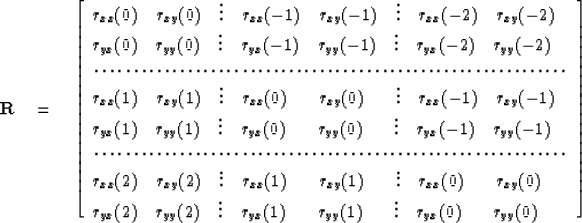(33)

We may take the 6 x 6 matrix of (33) and partition it into a 3 x 3 matrix of 2 x 2 submatrices. If we define the submatrix blocks as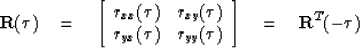(34)

then (33) in terms of the blocks defined in (34) is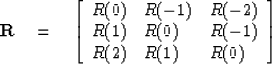(35)

The matrix in (35) is called block Toeplitz or multichannel Toeplitz. As with the ordinary Toeplitz matrix there is a trick method of solution. It will be taken up in the next section.

The reader should note that the matrix R does not depend on the desired output d. This results in a computational saving when there is more than one possible output. An example would be when it is desired to predict several different series or distances into the future on a given series.

## EXERCISES:

1. In the exercises of Chapter 2, we determined B(Z) and A(Z) such that some given power series C(Z) was expressed as C(Z) = B(Z)/A(Z). Write normal equations (do not solve them) for doing this in an approximate way by minimizing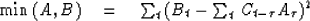where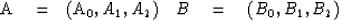subject to the constraint A0 - 1. (It can be proved that A(Z) comes out minimum-phase by examining the Levinson recursion.)Next: LEVINSON RECURSION Up: Waveform applications of least Previous: ADAPTIVE FILTERS
Stanford Exploration Project
10/30/1997